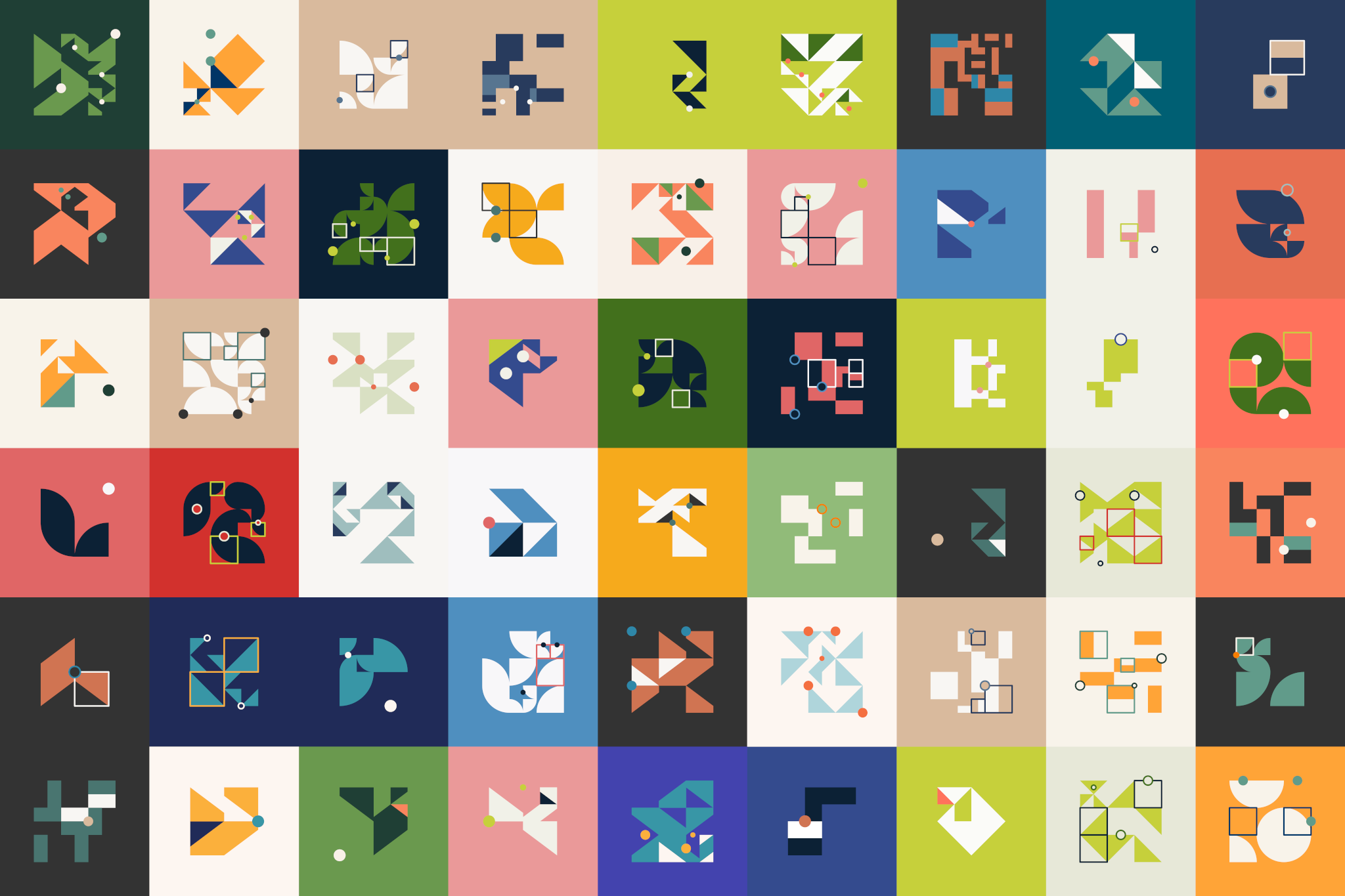# Generating Default Profile Icons for Saturn Cloud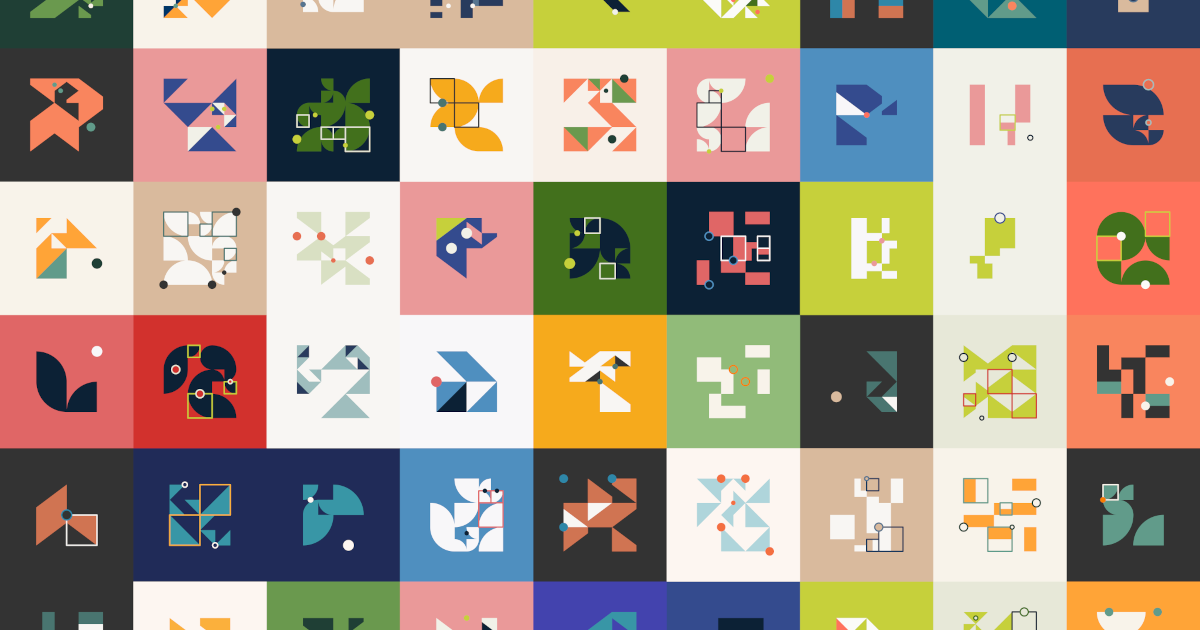In February, I was approached for a potential generative art project. Saturn Cloud wanted to create generative icons for users. The requirements were:

• The algorithm needed to be written preferably in R
• It needed to be a single function that takes in a username as the input and returns a PNG image
• The outputs should be diverse
• It shouldn’t have too many dependencies
• It shouldn’t take more than ~1 sec to run

## Inspiration

Due to the requirement of efficiency (~1 sec to run, oh my), I decided to go with a minimal generative system. Personally this meant I needed to avoid techniques in my personal toolkit such as circle packing/collision detection or recursive heavy techniques. As a side note, there are ways to have these techniques run very fast in R using rcpp, but I don’t program in C++. Fortunately, this left my one true love - grids.

Grids are something that I enjoy using in my work because 1) no matter how many shapes you pack or layer into the grid the piece will still retain an underlying symmetry, and 2) even simple grids allow a wide variety of outputs.

There was also the requirement that the outputs should be diverse. How this was presented to me was that if a Saturn Cloud employee needed to identify a user, in a group of user comments, they should be able to pinpoint the image quickly. This meant that visual diversity is important and I decided to address that using colors as well as a reasonable variety in shapes. However, it was also important to me for there to be a coherent style across images which is where a consistent layering technique came in.

## Composition of a Piece

Each output is composed of four “layers” that stack on top of each other to make a final piece:

1. Grid setup
2. Shapes
3. Squares
4. Circles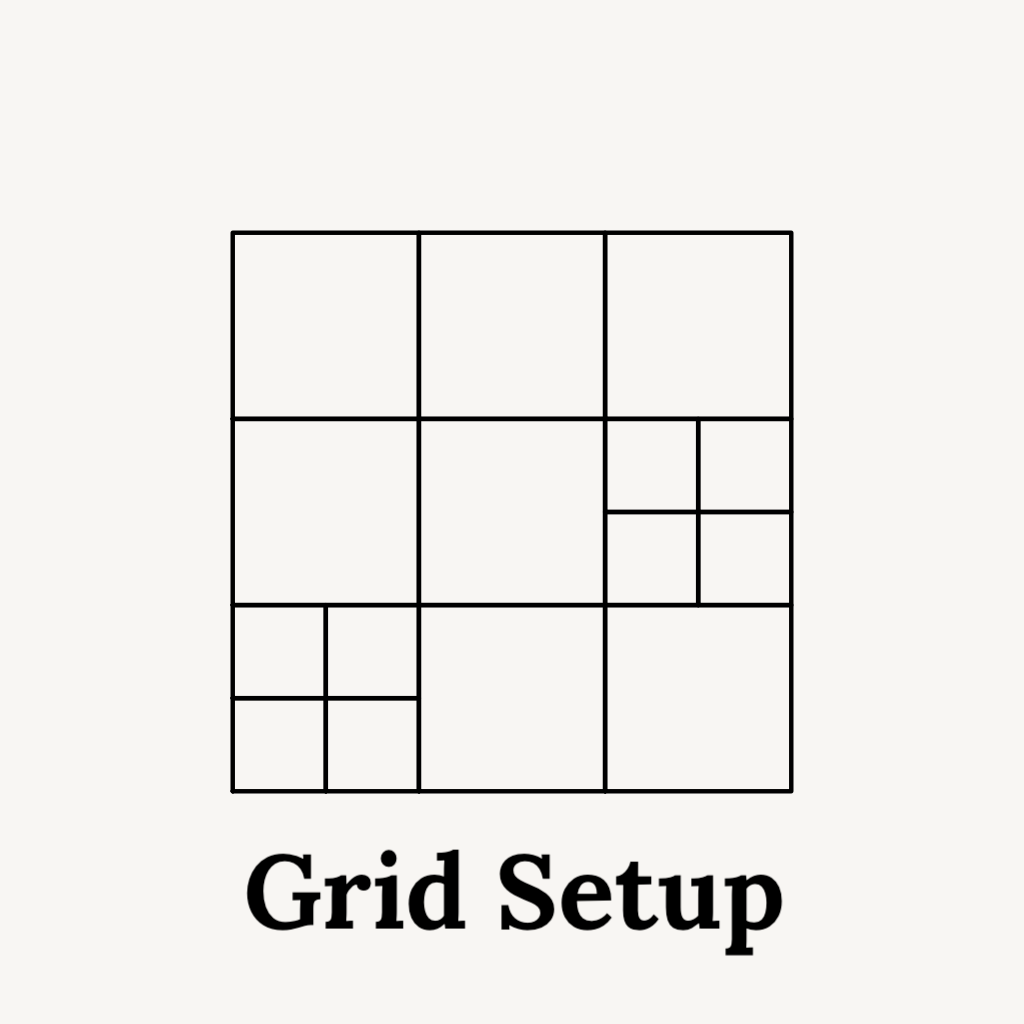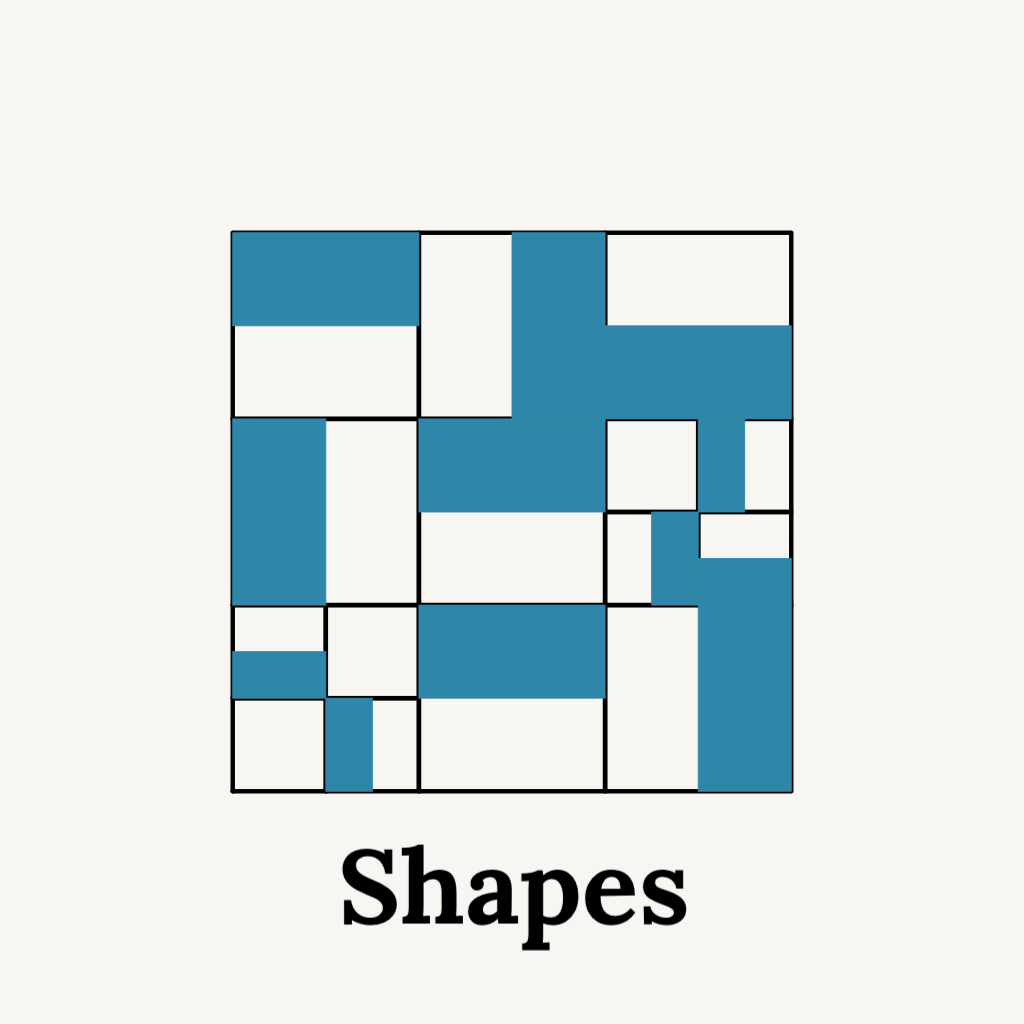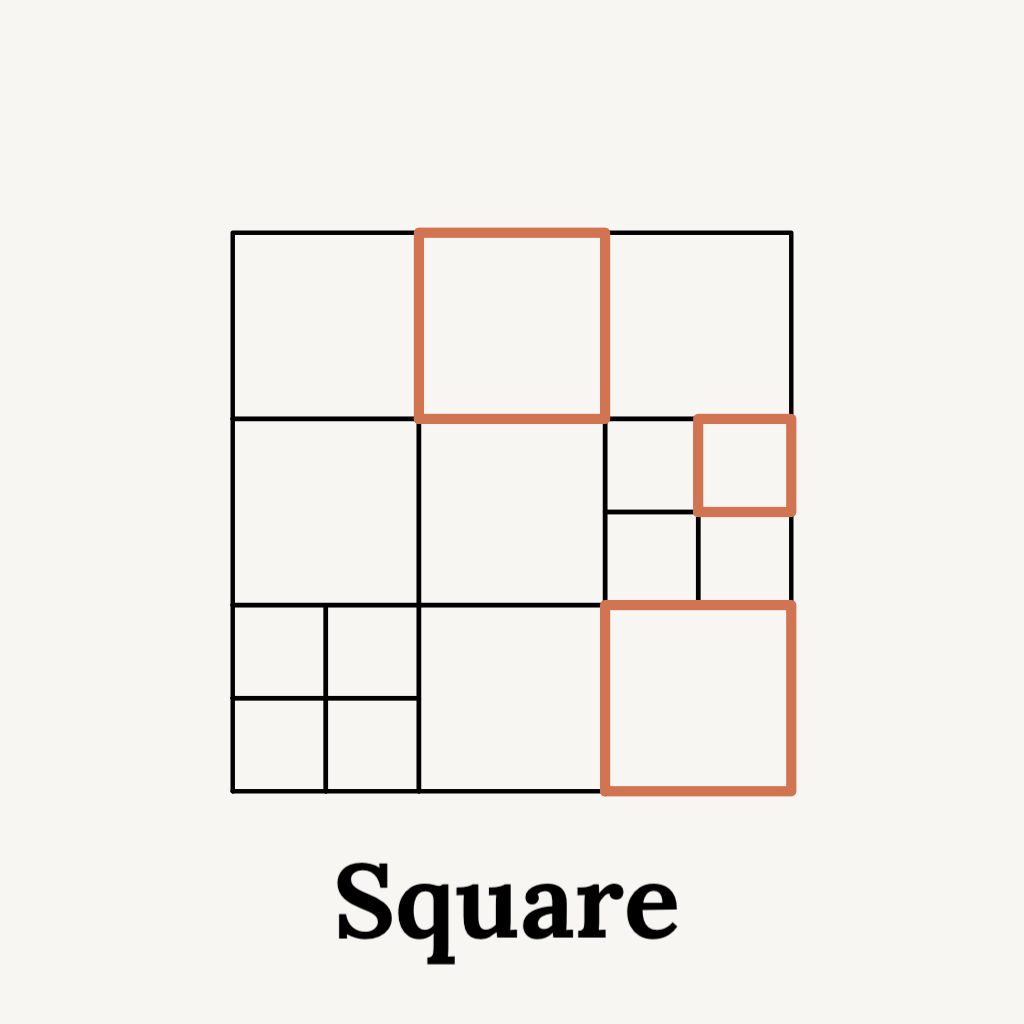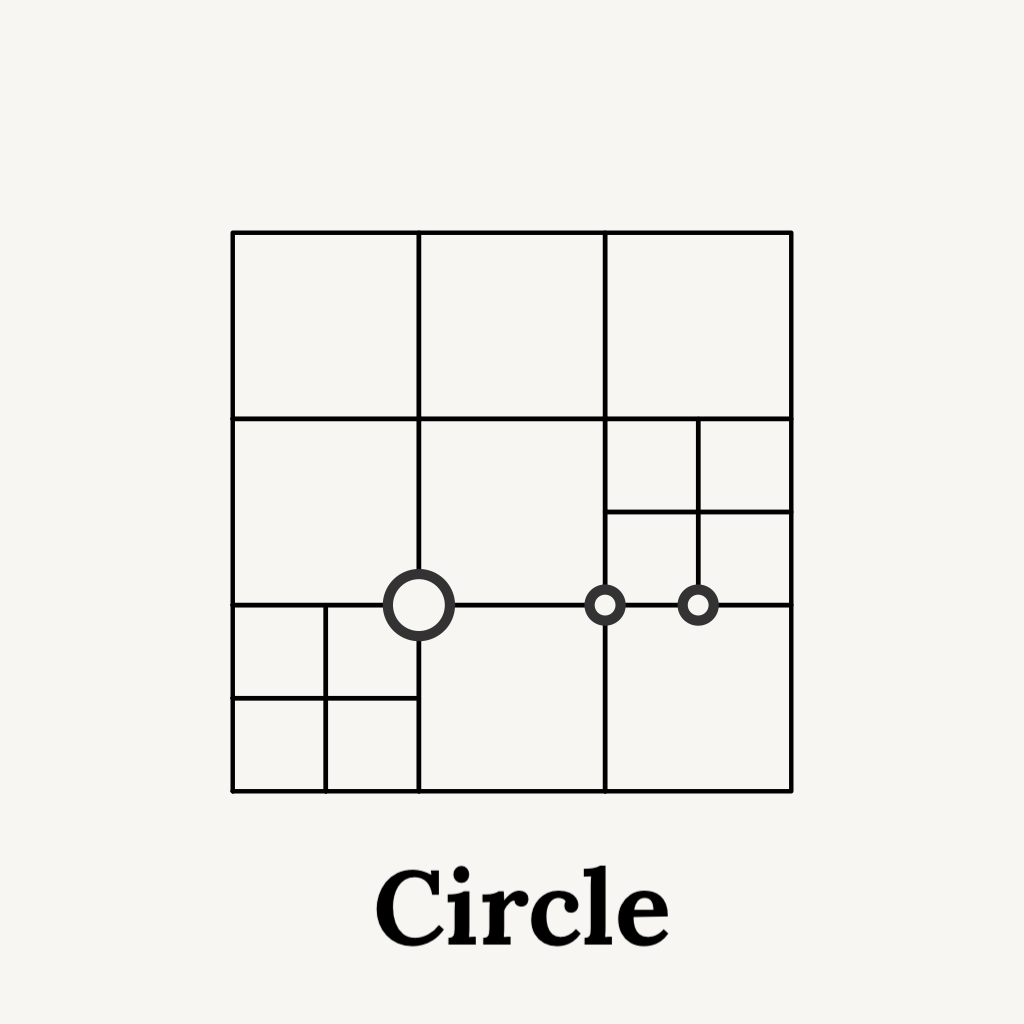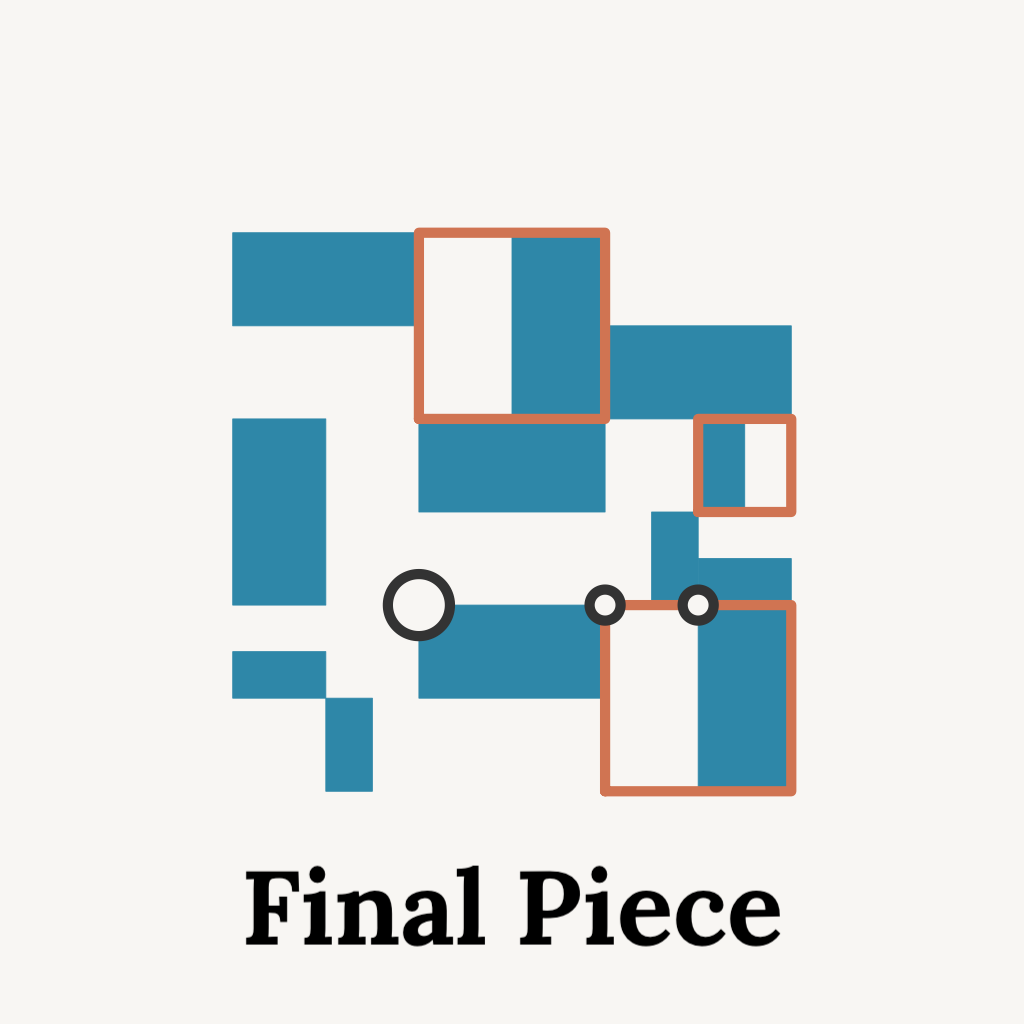## Aesthetics

### Grids

There are two grid options: 2x2 square or 3x3 square. Each square of the grid had a 15%/25% probability of being subdivided into a smaller 2x2 nested within the larger grid. This was to make it very rare (but not impossible!) for any grid to be completely subdivided. This grid layout was inspired by the layouts in Quadtrees.

The grid is the first layer because it is the anchor of the piece. Every subsequent layer builds off of the layout of the grid. This creates consistent structure in the composition of the piece even with each layer implementing various methods of randomness. It also makes it more seamless to build the piece, since you do not need to constantly recalculate a location for the elements in each layer.

How to create a grid

There are many ways to create a square grid but here is my go-to method and the method implemented in the system.

First, I set up my parameters. I want a 3x3 grid where each square has a width and height of 5 and the probability of a square subdividing is 15%.

``````width = 5
grid_options = 3
grid_subdiv_prob = c(0.85, 0.15)
``````

In my mental model of a square, the vertices always start counterclockwise, from the bottom left as picture below. I always build a grid using the bottom left vertex.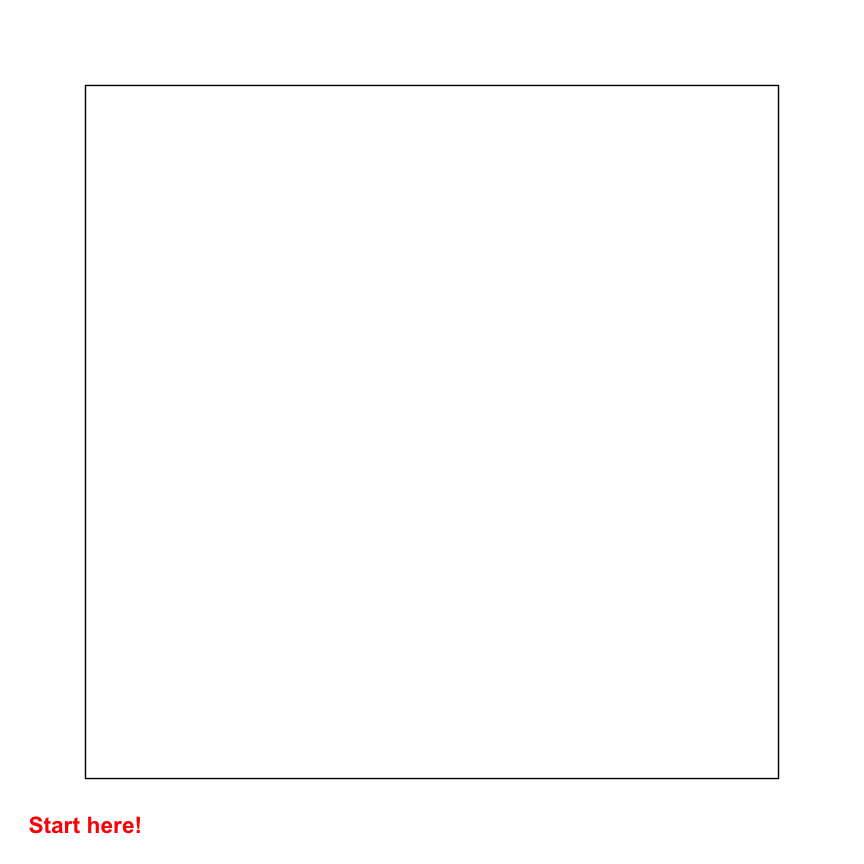When creating the grid, I use expand.grid() which creates a dataframe of all combinations of the potential bottom left coordinates. Then using sample, each square can be selected for subdividing (setting subdivide == 1).

``````grid = expand.grid(
x = seq(0,
by = width,
length.out = grid_options),
y = seq(0,
by = width,
length.out = grid_options))

grid\$subdivide = sample(0:1,
size = nrow(grid),
replace = TRUE,
prob = grid_subdiv_prob)
``````

Before we actually implement the subdivision, I need to introduce build_square_grid().

This function takes in the bottom left vertices, the width of each grid square, and whether the grid square has been selected for subdivision. If it has been selected, it turns the bottom left vertex into 4 additional smaller vertices and updates the width to be a quarter of the original width. Therefore in the place where one large square would be, there are four smaller squares.

``````build_square_grid = function(x0, y0, width, subdivide){
if(subdivide == 1){
dplyr::tibble(
x = c(x0, x0 + width/2, x0 + width/2, x0),
y = c(y0, y0, y0 + width/2, y0 + width/2),
width = width/2
)

} else if(subdivide == 0){
dplyr::tibble(
x = x0,
y = y0,
width = width
)
}
}
``````

Then comes the fun part of using map_dfr(), my most used function in the purrr package, to iterate through each row of the grid and when a bottom left vertices is selected for subdivision, expanding the vertices into 4 additional vertices.

``````subdiv_grid =
purrr::map_dfr(
1:nrow(grid),
~build_square_grid(
x0 = grid\$x[.x],
y0 = grid\$y[.x],
width = width,
subdivide = grid\$subdivide[.x]))
``````

Below is the location of the bottom left point for each square in the grid created in red, and the larger square that could arise from each point in black. You can see that the center square was subdivided into smaller squares.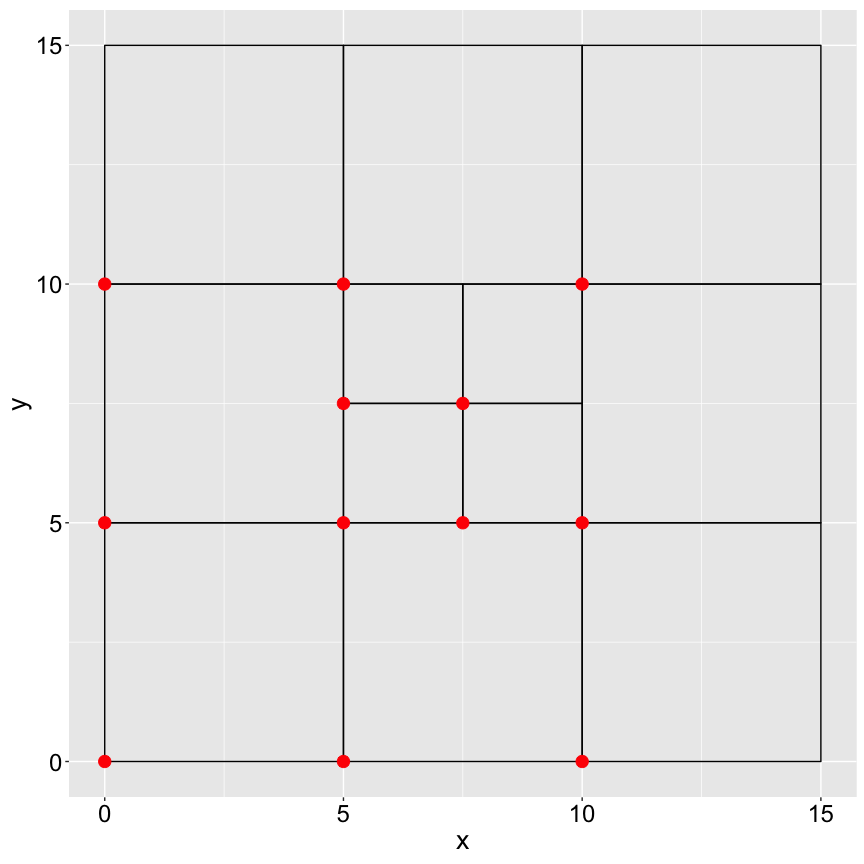### Shapes

There are four shape options in this system: right triangles, rectangles, jittered rectangles, and quarter circles. Each shape has five appearance options - four are different orientations within the grid and one is the shape not appearing. Each shape has a repeating pattern throughout the piece that is randomly generated. The pattern repeats as you travel first horizontally (from left to right) then vertically (from bottom to top).

I went for these shapes because they are easy to create thus quick to generate, but most importantly, they always touched at least three sides of each square. When exploring potential shapes, the ones that covered the space of the grid, but left a hint of negative space were the most visually appealing to me.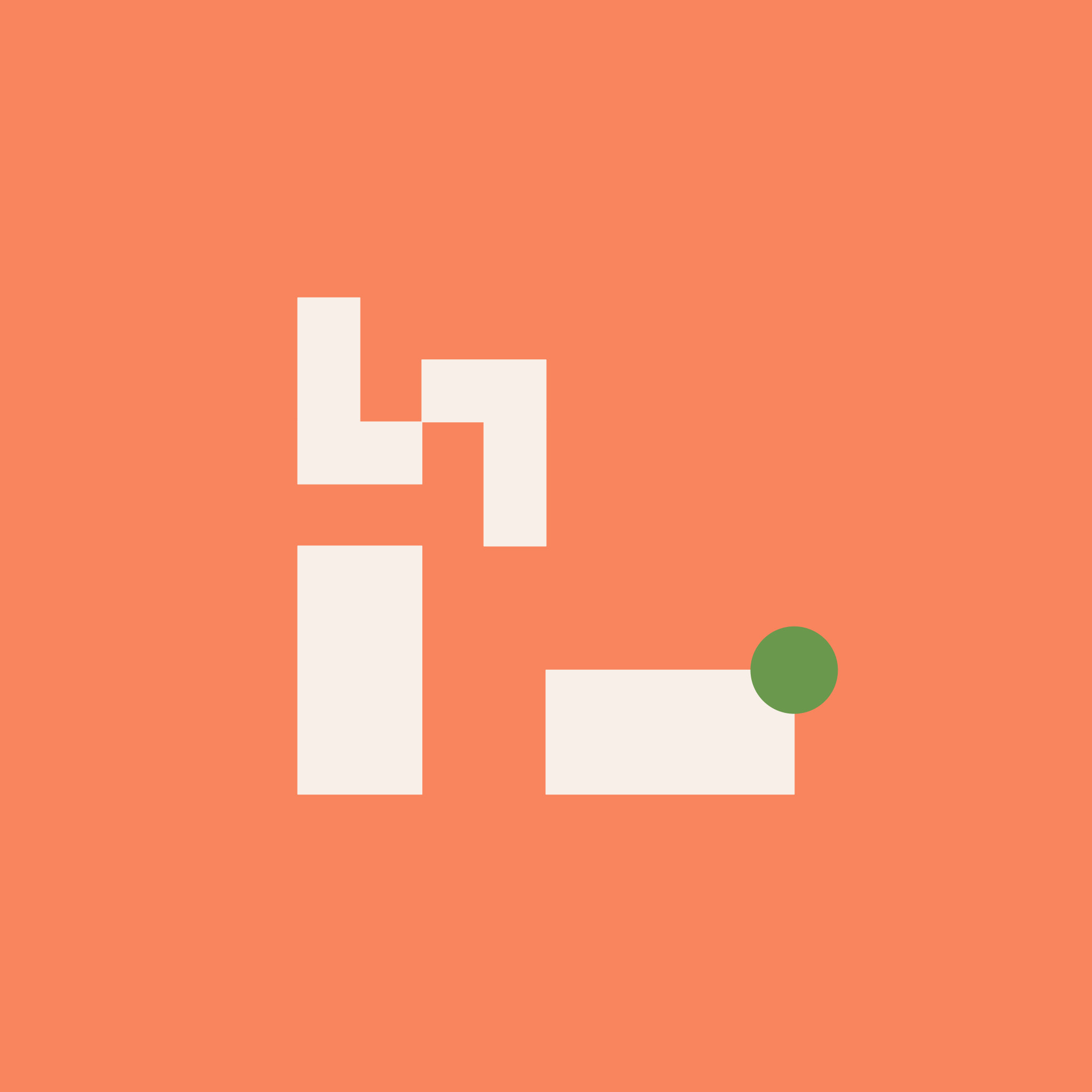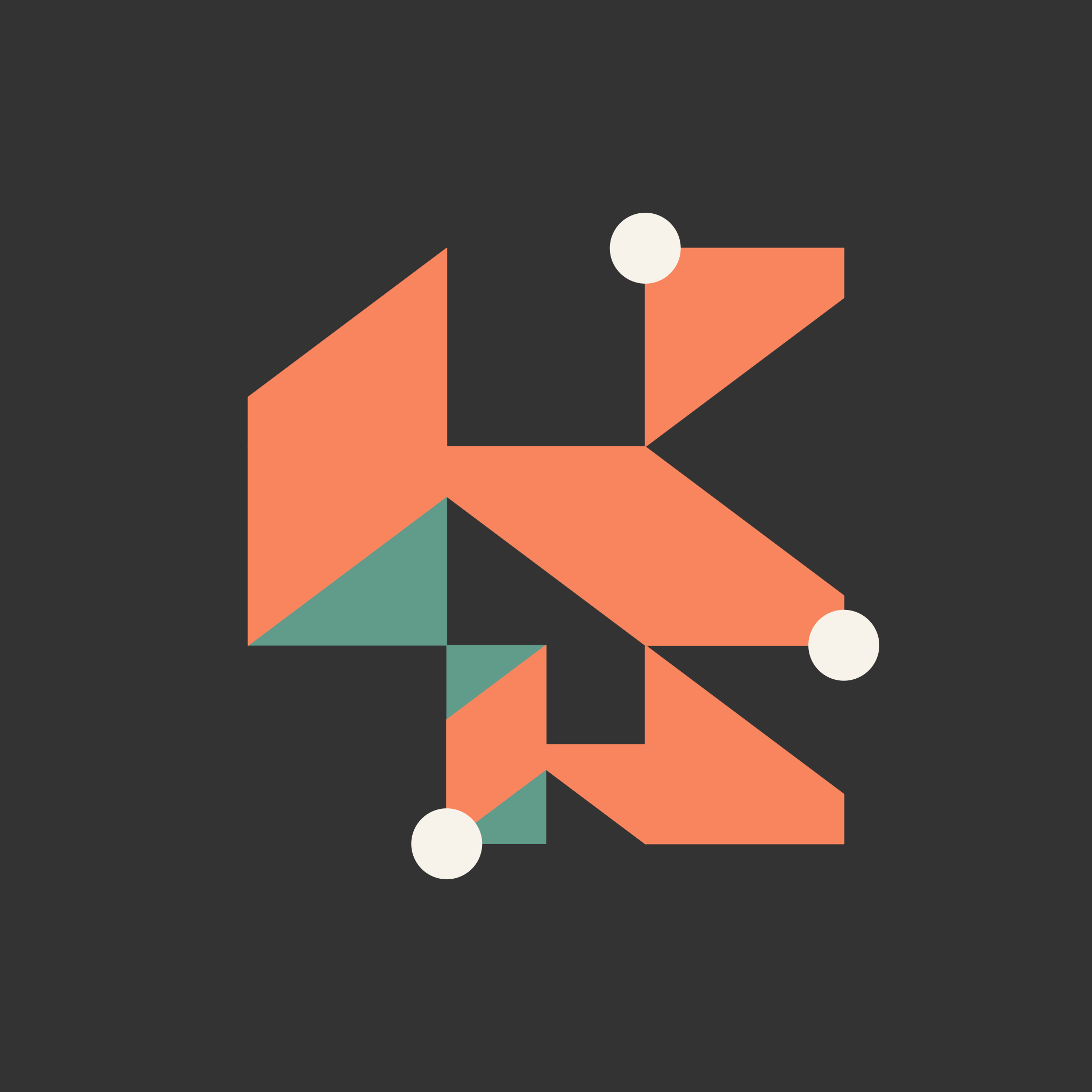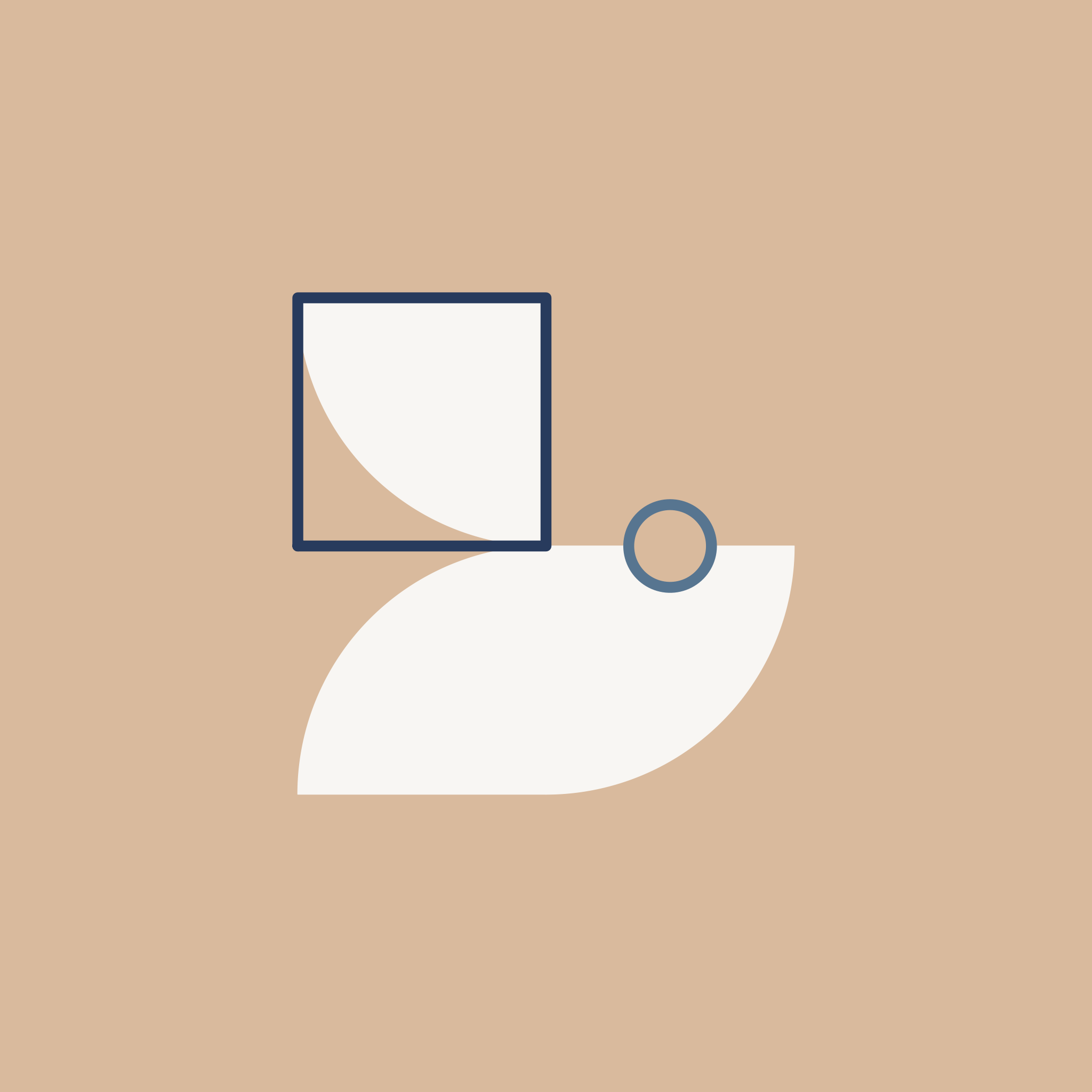Figure: Outputs featuring shapes of right triangles, rectangles, jittered rectangles, and quarter circles

### Squares

The square layer gives a little peak at the underlying structure of the piece, the grid. To do this, up to 1/4 of the grid squares can be sampled to be visualized. This allowed the square to be a part of most outputs, but not the star (the star is the shapes!).

Within one output the squares can either be filled, an outline, or no squares are shown. If the square is filled, it is layered underneath the shape layer. If the square is an outline, it is layered ontop of the shape layer. There is a slightly higher probability of the square being filled than appearing as an outline.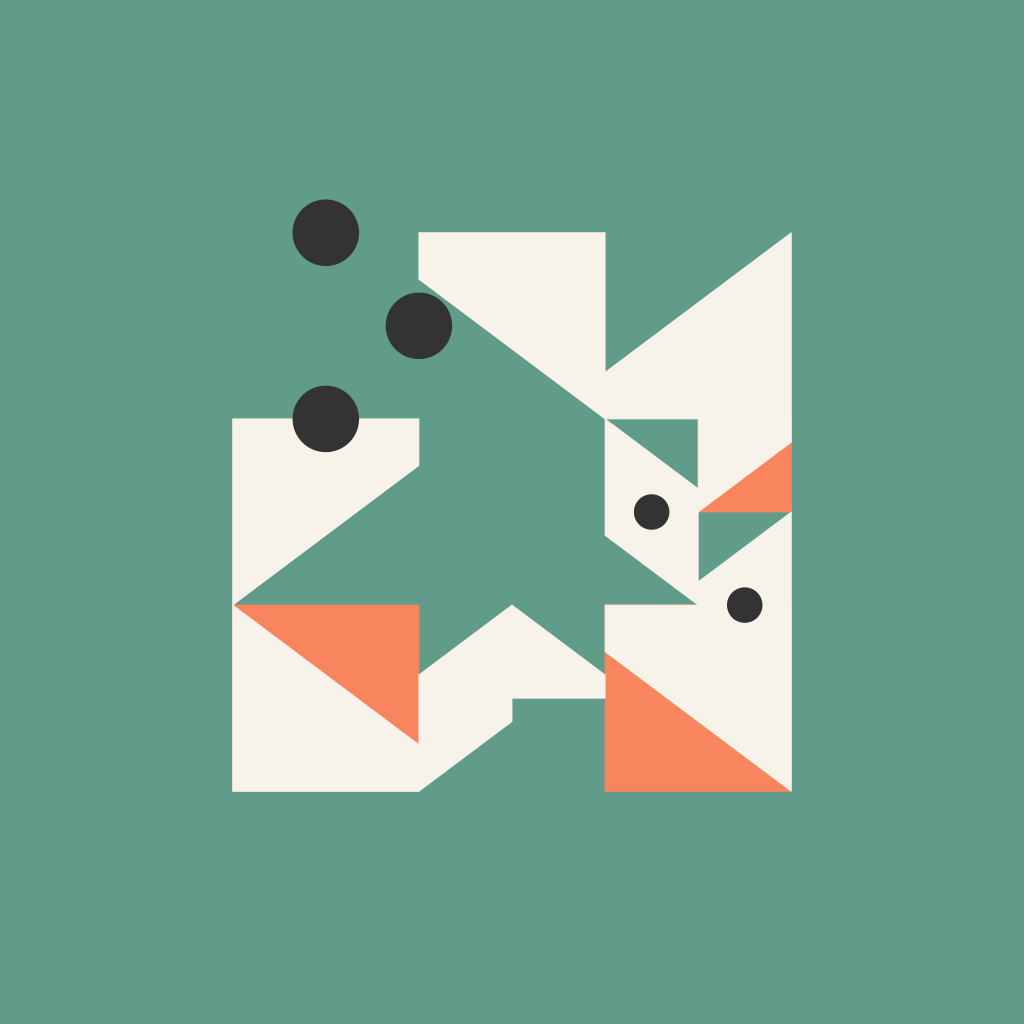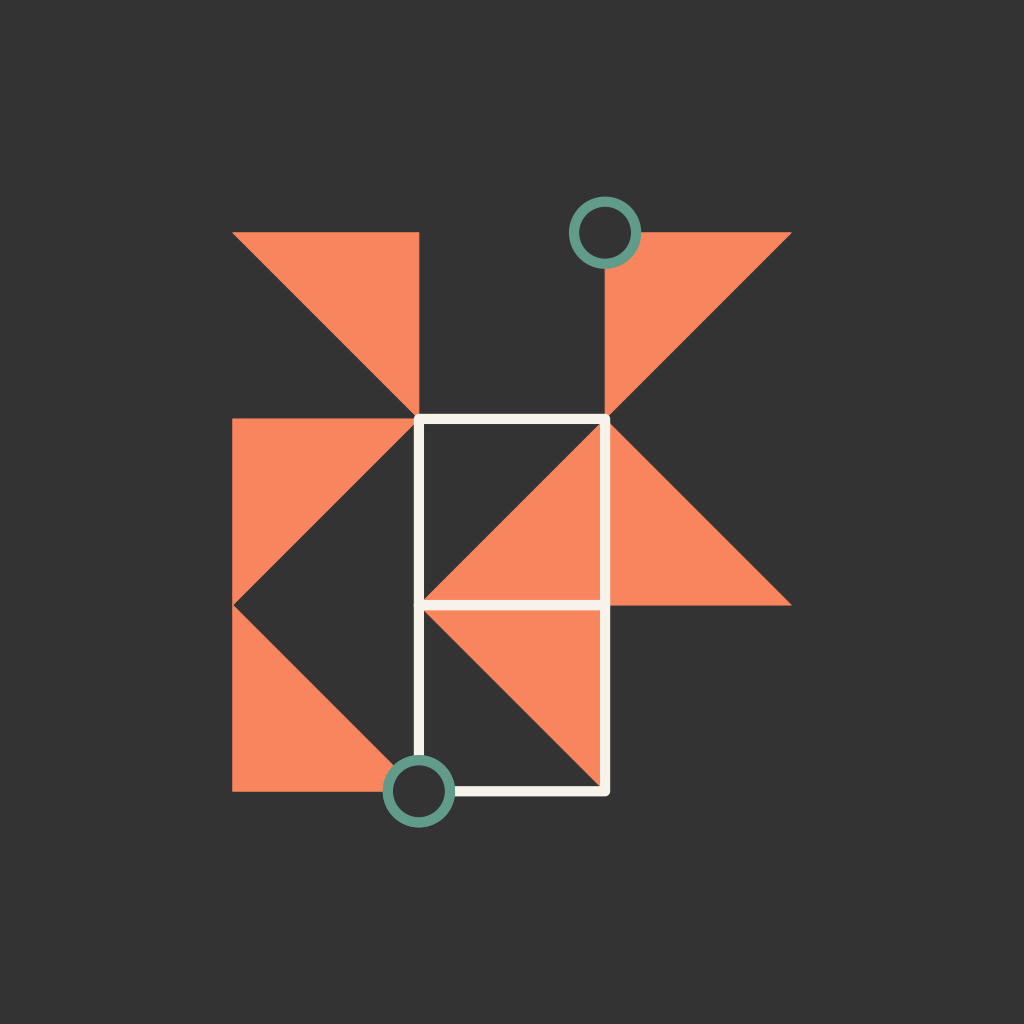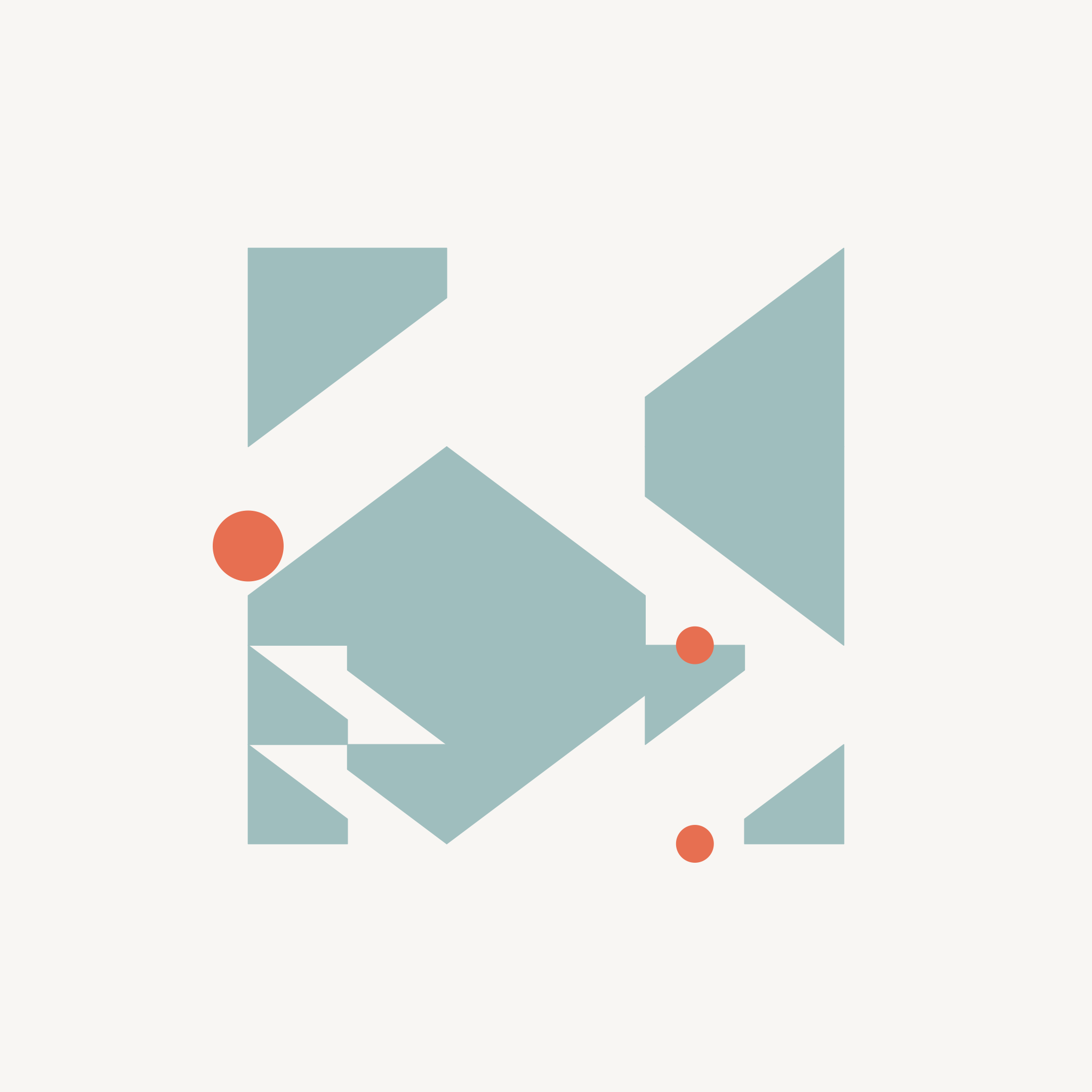Figure: Three outputs, the leftmost output has squares that are filled and layered underneath the shapes. The middle output has squares that are outlines and layered on top of the shapes. The rightmost output has no squares.

### Circles

There are two main parameters for the circles - where they are placed and how they appear.

The circles can either be placed at 1) an intersection of two or more grid squares, 2) the midpoint between two vertices of the same grid square, or 3) there can be no circles. Again this placement is intentional. Complete randomness in placement would be chaotic (which honestly can be fun but was not what I was looking for!). Instead, placing them at intersections or midpoints gives, again, visual cues to the underlying structure of the grid. No more than 35% of midpoints or intersections can be sampled for the placement of a circle.

Similarly to squares, circles can either be filled or an outline. The radius of the circle is also proportional the width of the square grid it is placed on.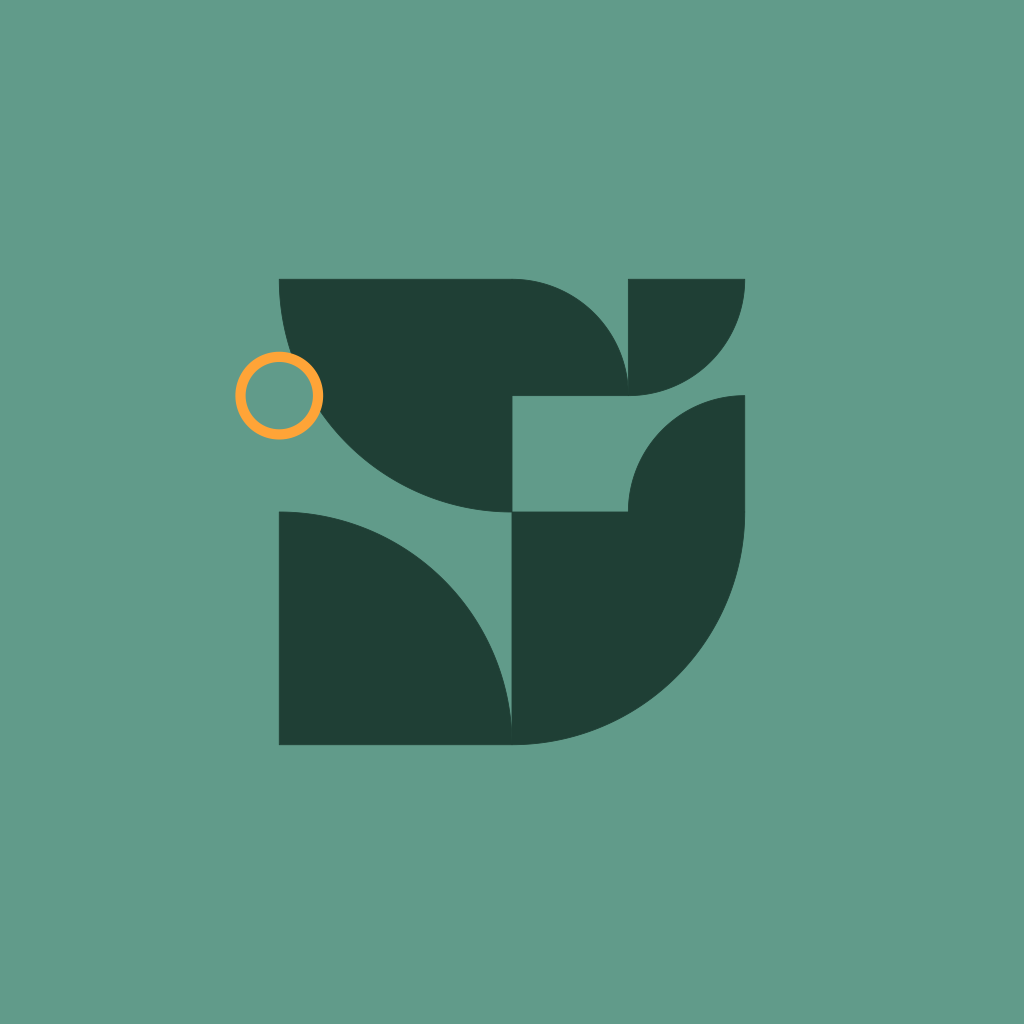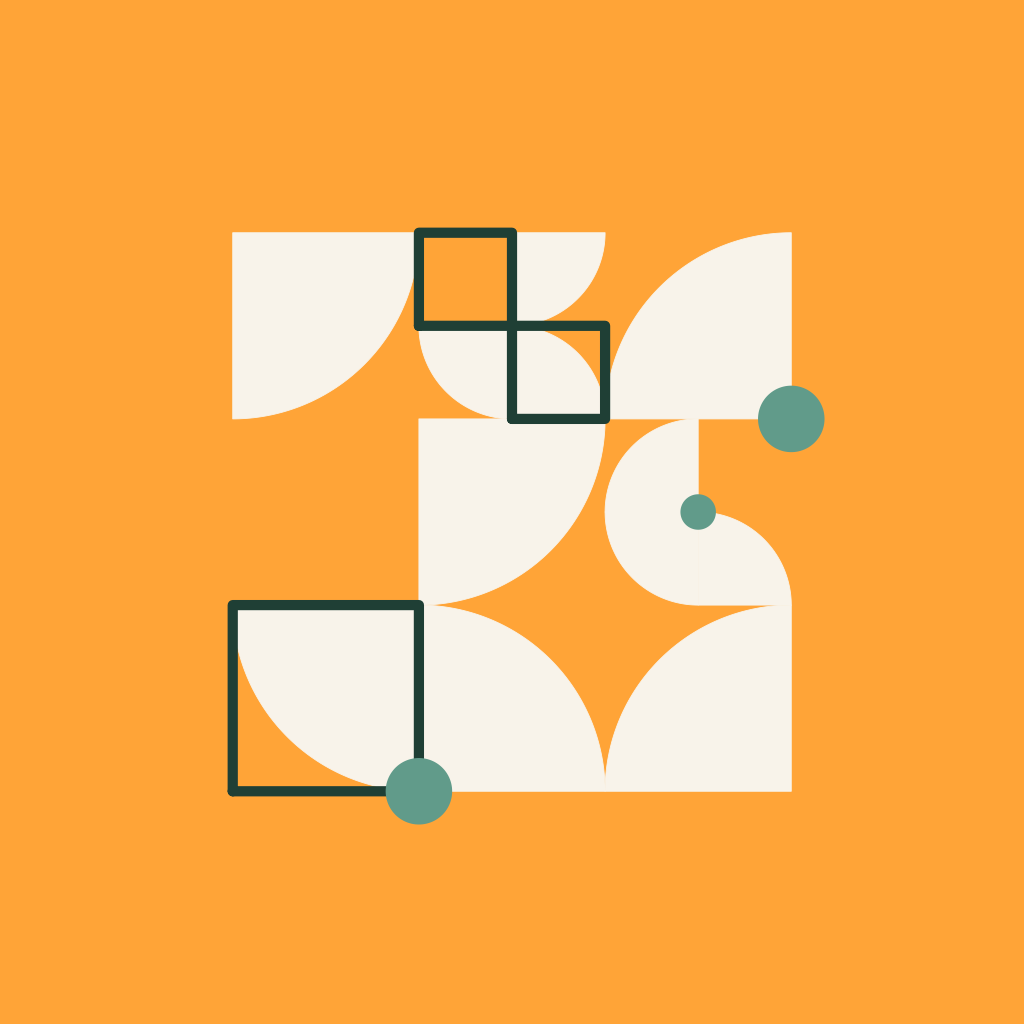Figure: Two outputs with the same color palette and shape selection. The left output has a circle that is filled and placed at a midpoint. The right output has a circle that is an outline and placed at intersections.

Now that I’ve introduced the circle layer, I can walk us through how we can use the earlier grid to add circles to our piece. I’ll specifically show an example of placing circles at midpoints.

How to add circles to grid

First, we will set up our parameters. This is an easy one and we are only setting the percentage of circles we want as 25%.

``````perc_circles = .25
``````

To create circles, we will need two functions: circle_util() and create_midpoint_circles().

circle_util() is a helper function that takes in the x, y, and width and performs some simple trig to create the points of a circle (another useful function to do this is ggforce::geom_circle, but I was trying to limit my dependencies in this system).

create_midpoint_circles() uses circle_util() to then create circles at the various midpoints along each grid square. Type 1 places the circle between the bottom left and bottom right vertices, type 2 places the circle between the bottom right and top right vertices, and so forth.

``````circle_util = function(x0, y0, width) {
tau = seq(0, 2 * pi, length.out = 100)

dplyr::tibble(x = x0 + sin(tau) * radii,
y = y0 + cos(tau) * radii)
}

create_midpoint_circles = function(x0, y0, width, type) {
if (type == 1) {
circle_util(x0 = x0 + width / 2,
y0 = y0,
width = width)
} else if (type == 2) {
circle_util(x0 = x0 + width,
y0 = y0 + width / 2,
width = width)
} else if (type == 3) {
circle_util(x0 = x0 + width / 2,
y0 = y0 + width,
width = width)
} else if (type == 4) {
circle_util(x0 = x0,
y0 = y0  + width / 2,
width = width)
}

}
``````

We then sample 25% of the row numbers of the subdiv_grid we created earlier without replacement. Using circle_sample as index numbers, we create a new dataset from subddiv_grid that is the sampled rows for circle_grid.

Then, again, map_dfr() my favorite purrr function comes in to iterate through circle_grid and create circles at the sampled midpoints from the grid.

``````circle_sample = sample(
1:nrow(subdiv_grid),
size = nrow(subdiv_grid) * perc_circles,
replace = FALSE
)

circle_grid = subdiv_grid[circle_sample,]

circle_layer =
purrr::map_dfr(1:nrow(circle_grid),
~dplyr::bind_cols(
id = .x,
create_midpoint_circles(
x0 = circle_grid\$x[.x],
y0 = circle_grid\$y[.x],
width = circle_grid\$width[.x],
type = sample(1:4, size = 1)
)
))
``````

Below is the grid from before, but with the sampled circles added.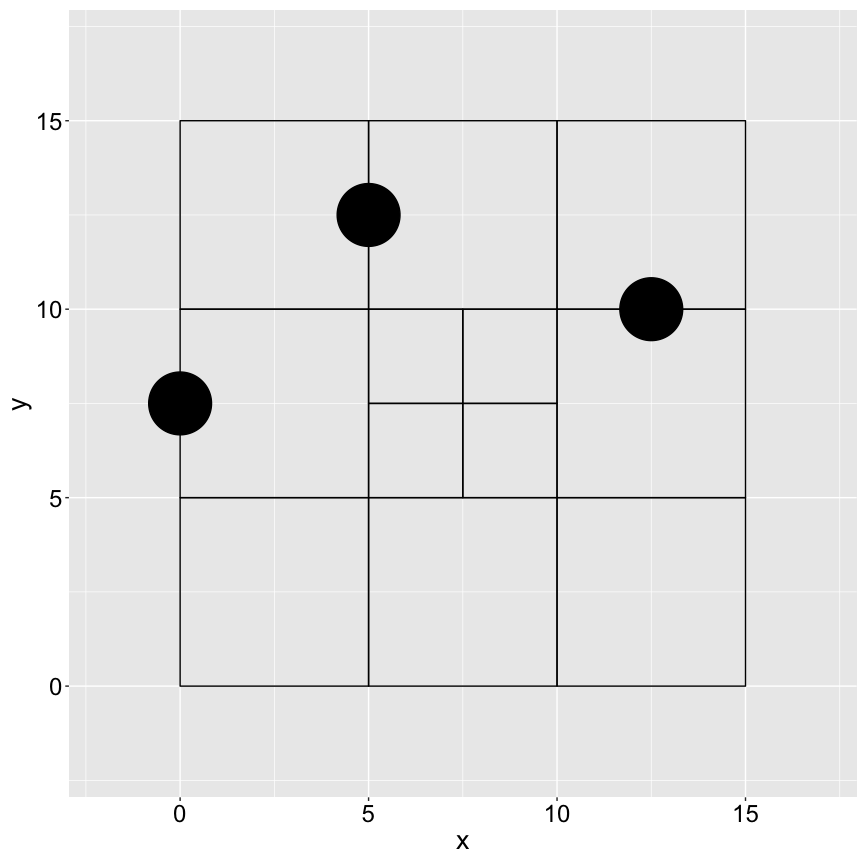### Palettes

My color selection method is probably what went through the most iterations when creating this system. The first major difficulty for me was figuring out how to preventcolors that are too similar from appearing in the same output. While I love a monotone moment, a sea icons where every single element is green would not be helpful to the Saturn Cloud employees. The second major difficulty was figuring out how to make sure there was enough contrast to easily distinguish between the differen layers in the output at various image size dimensions.

To do this, I decided to go with four parent palettes: Soft, Saturn, Summer, and Shock. Each parent palette is broken into sub-palettes to balance out color diversity and contrast. All sub-palettes have an equal probability of being selected, but are specifically built such that certain colors are always grouped together.

Some examples from each palette:

##### Saturn##### Shock##### Soft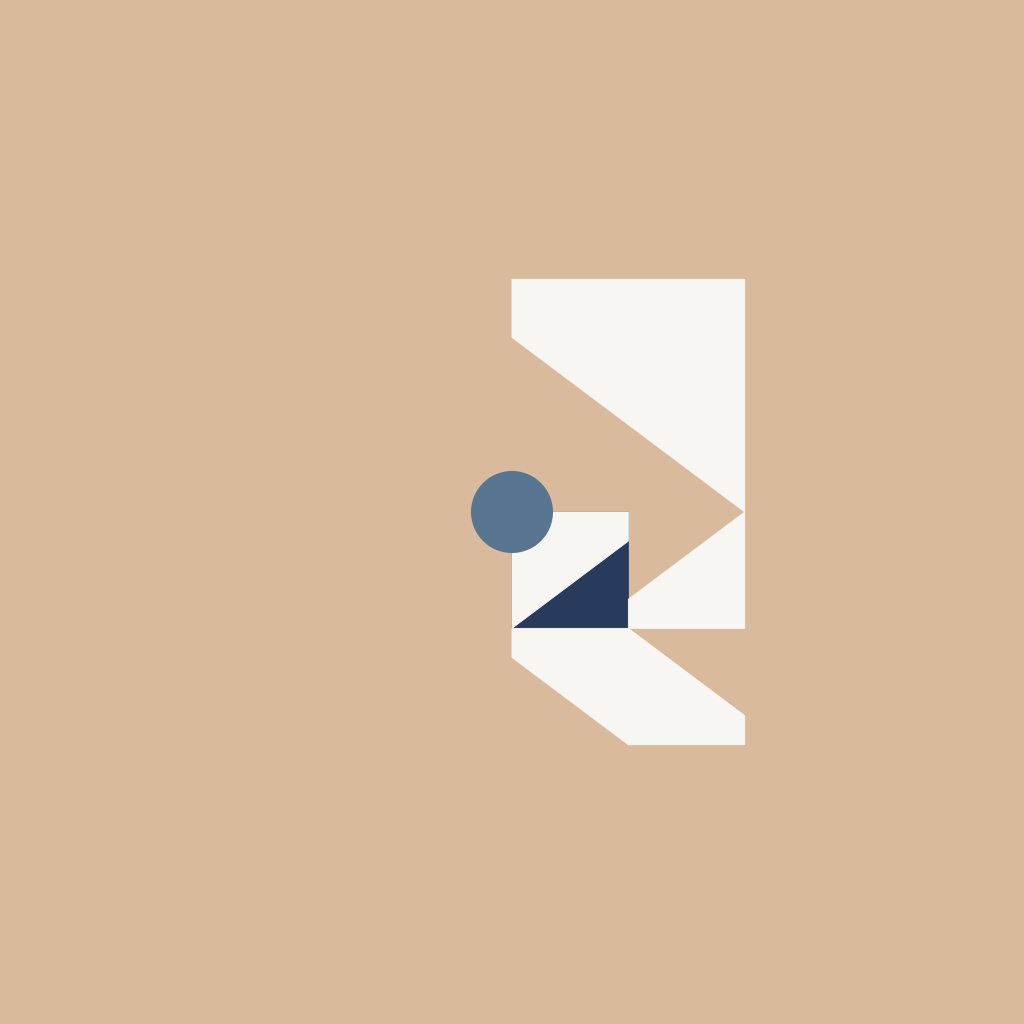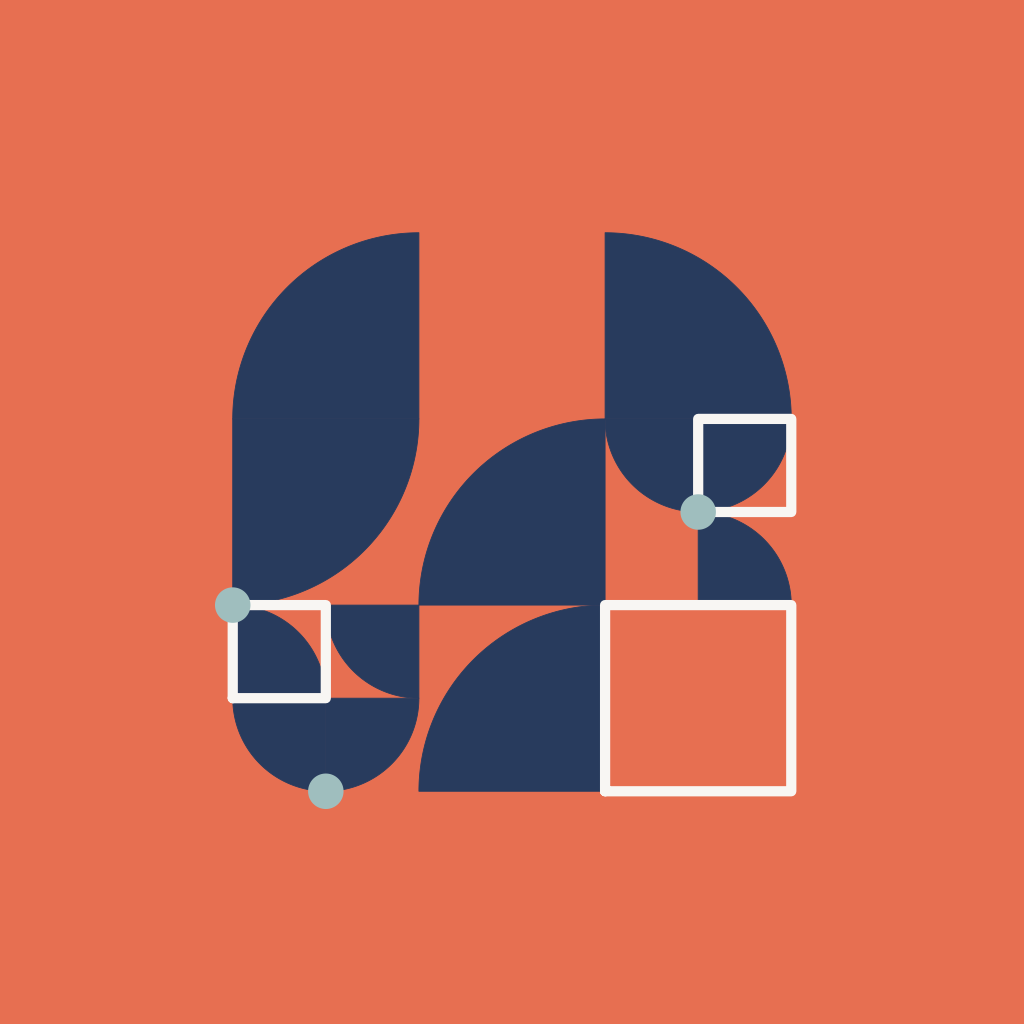##### Summer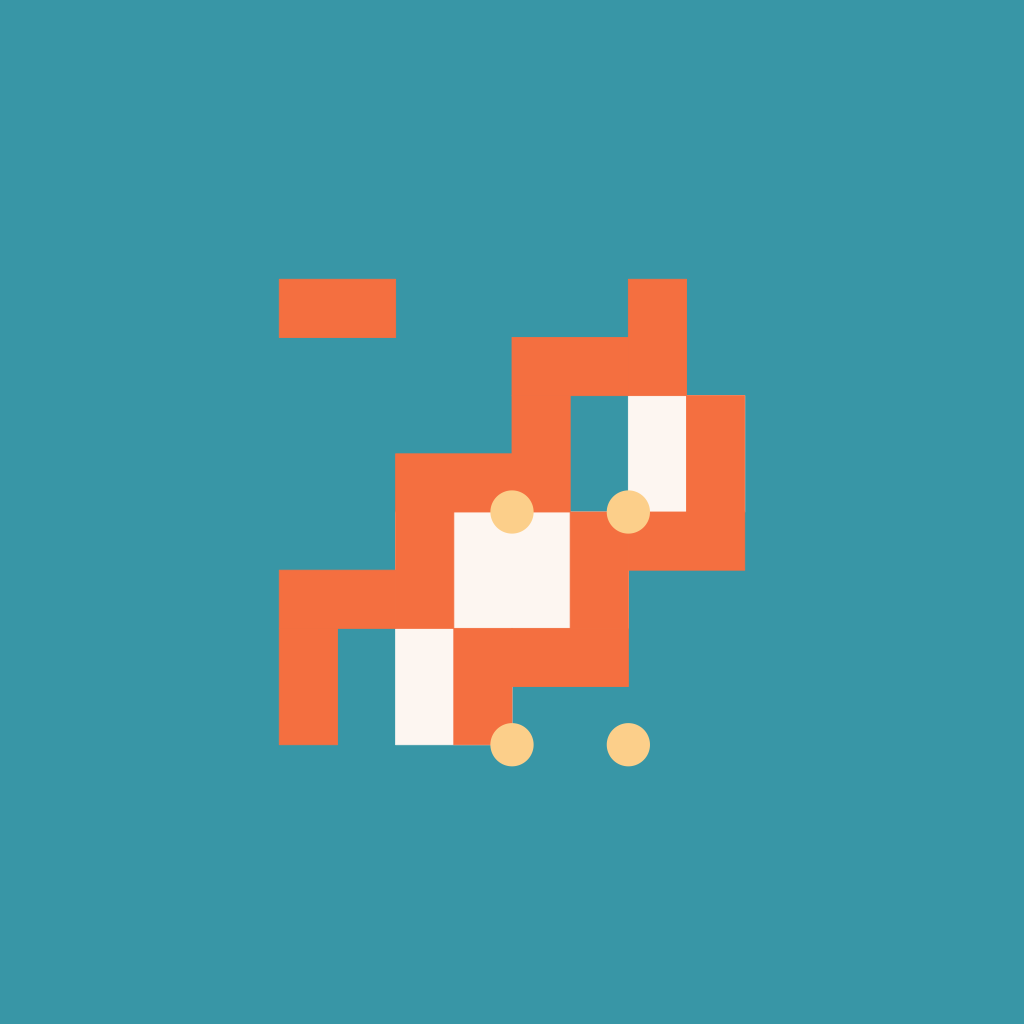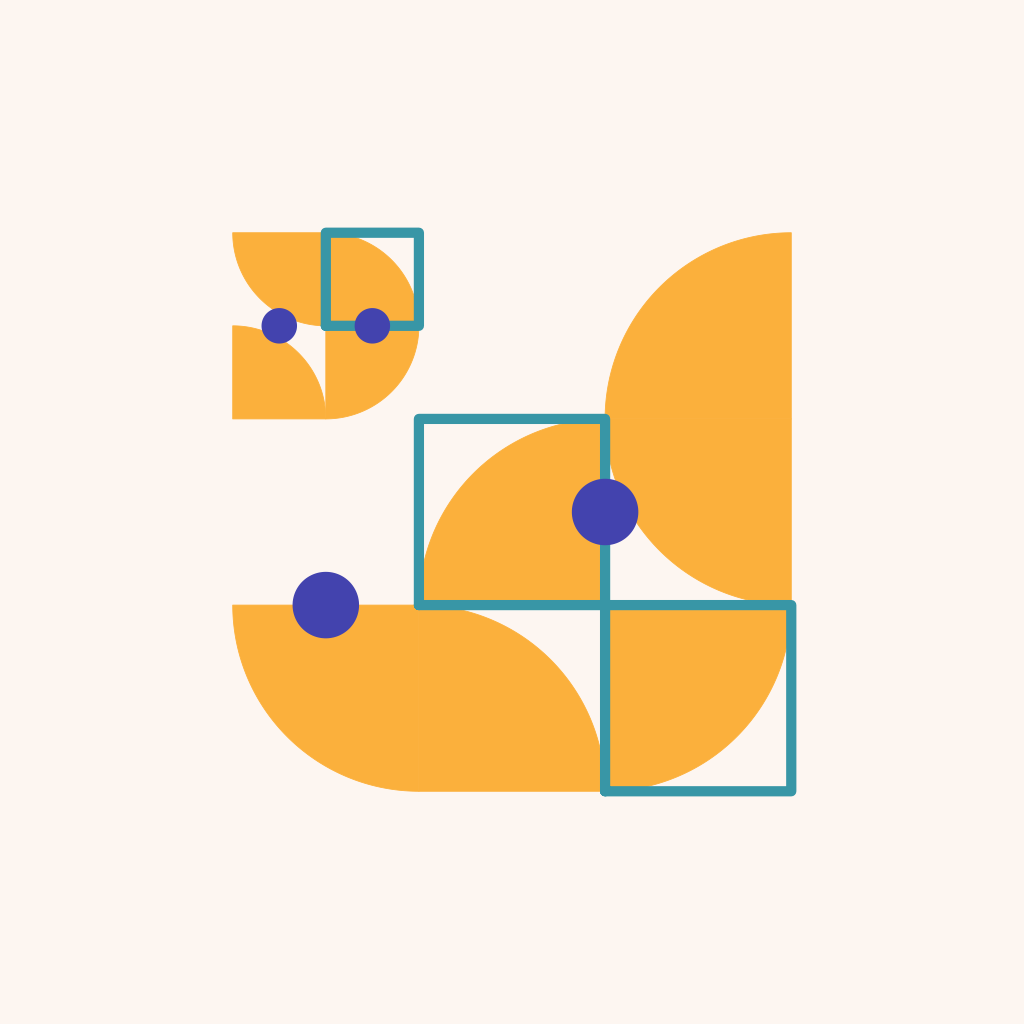## Final

And this is the thought process behind my creation of a generative system for profile pictures for Saturn Cloud! Thanks so much to Saturn Cloud for hiring me for this project, it was a ton of fun. As a last hoorah - here is a collage of some outputs that I enjoyed and randomly generated.